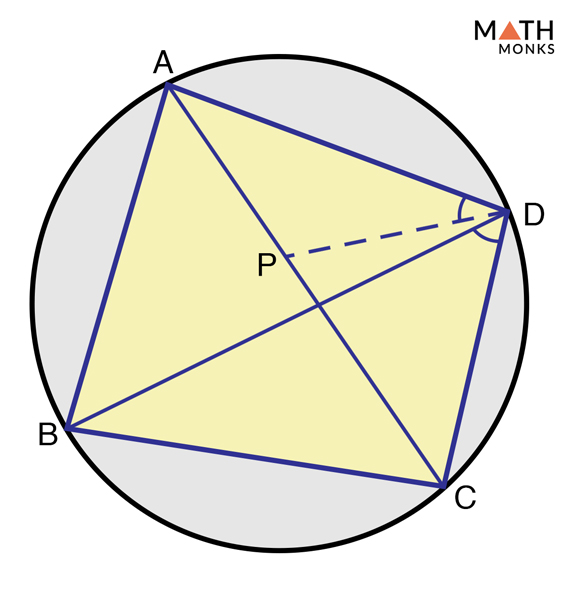## Definition

A cyclic quadrilateral is a quadrilateral with all its four vertices or corners lying on the circle. It is thus also called an inscribed quadrilateral. When any four points on the circumference of a circle are joined, they form the vertices of a cyclic quadrilateral.

## Properties

1. Has four vertices that lie on the circumference of a circle. The four vertices make four angles; in a cyclic quadrilateral, ABCD, A, B, C, D are the vertices making angles ∠DAB, ∠ABC, ∠BCD, and ∠CDA
2. Opposite angles add up to 180°; so ∠DAB + ∠BCD = 180° and ∠ABC + ∠CDA = 180°
3. The exterior angle formed when any one side is extended is equal to the opposite interior angle; ∠DCE = ∠DAB

## Formulas

### Angles

In a cyclic quadrilateral, the sum of the opposite angles is 180°. In other words, the pair of opposite angles in a cyclic quadrilateral is supplementary. The formula is given below:

In the cyclic quadrilateral ABCD, find ∠DAB and ∠ABC when ∠BCD = 110° and ∠CDA= 70°.

Solution:

As we know,
∠DAB + ∠BCD = 180° and ∠ABC + ∠CDA = 180°, here ∠BCD = 110° and ∠CDA= 80°
Now, ∠DAB + 110° = 180°
∠DAB = 180° – 110°
= 70°
Similarly,
∠ABC + 80° = 180°
∠ABC = 180° – 80°
= 100°

### Diagonals

They are the line segments joining opposite vertices or corners of the cyclic quadrilateral. There are two diagonal (d1 and d2) in a cyclic quadrilateral. The formula is given below:

Find the diagonals of a cyclic quadrilateral whose sides are 3 m, 4 m, 6m, and 8 m.

Solution:

As we know,
Diagonal (d1) = √(ac + bd) (ad + bc)/ab +cd
= √[(3 x 6) + (4 x 8)] [(3 x 8) + (4 x6)]/[(3 x 4) + (6 x 8)] m
= √[(18 + 32) (24 + 24)]/[12 + 48] m
= √2400/60 m
= √40 m
= 6. 3245 m
Similarly,
Diagonal (d2) = √(ac + bd) (ab + cd)/ad + bc
= √[(3 x 6) + (4 x 8)] [(3 x 4) + (6 x 8)]/ [(3 x 8) + (4 x 6)] m
= √[(18 + 32) (12 + 48)]/[24 + 24] m
= √3000/48 m
= √62.5 m
= 7.9056 m

Also known as circumradius, it is a straight line drawn from the center to the circumference of the circle in a cyclic quadrilateral. Parameshvara’s formula for finding circumradius is given below:

Find the circumradius of the cyclic quadrilateral ABCD with center O whose sides measure 2 m, 4 m, 6m, and 10 m.

Solution:

As we know,
Circumradius (R) = 1/4√(ab + cd) (ac + bd) (ad + bc)/ (s-a) (s-b) (s-c) (s-d), here a = 2 cm, b = 4 cm, c = 6 cm, and d = 10 cm
In this cyclic quadrilateral, s = ½ (2 cm + 4 cm + 6 cm + 10 cm) = 11 cm
Since, R = 1/4√(ab + cd) (ac + bd) (ad + bc)/ (s-a) (s-b) (s-c) (s-d)
= 1/4√[{(2 X 4) + (6 X 10) } {(2 X 6) + (4 x 10)} {(2 x 10) + (4 x 6)}]/[(11 – 2)(11 – 4)(11 – 6)(11 – 10)] cm
= 1/4√[{8 + 60} {12 + 40} {20 + 24}]/[9 x 7 x 5 x 1] cm
= 1/4√1,55,584/315 cm
= 1/4√493.9174 cm
= 5.556 cm

### Area

It is the total space enclosed by the cyclic quadrilateral.  Brahmagupta’s formula for finding the area is given below:

Find the area of a cyclic quadrilateral whose sides are 6 cm, 8 cm, 10 cm, and 12 cm.

Solution:

As we know,
Area (A) = √(s-a) (s-b) (s-c) (s-d), here a = 2 cm, b = 4 cm, c = 6 cm, and d = 8 cm
In this cyclic quadrilateral, s = ½ (2 cm + 4 cm + 6 cm + 8 cm) = 10 cm
Since, A = √(s-a) (s-b) (s-c) (s-d)
= √(10-2)(10-4)(10-6)(10-8) cm2
=19. 5959 cm2

## Theorems

There are two important theorems that prove a cyclic quadrilateral. They are given below with their mathematical proofs.Prove that opposite angles of a cyclic quadrilateral are supplementary

To prove:

∠ABC + ∠CDA = 180° and ∠DAB + ∠BCD = 180°

Proof:

Let ABCD be a cyclic quadrilateral and ‘O’ be the center of the circle.
Join OB and OD.
a) ∠DAB = ½ ∠BOD (By theorem: The angle extended by an arc at the center is double the angle on the circle)
b) Similarly, ∠BCD = ½ reflex ∠BOD
c) Again, ∠DAB + ∠BCD = ½ reflex ∠BOD + ½ reflex ∠BOD
Adding (a) and (b) we get,
∠DAB + ∠BCD = ½(∠BOD + reflex ∠BOD)
∠DAB + ∠BCD = ½ x 360°
∠DAB + ∠BCD = 180°
Similarly,
∠ABC + ∠CDA = 180°
Hence Proved

### Ptolemy’s TheoremProve Ptolemy’s Theorem

To prove:

AC x BD = AD x CB + DC x AB

Proof:

Let ABCD be a cyclic quadrilateral, and ‘P’ be a point on the cord AC such that ∠ADP = ∠CDB
Adding (a) and (b) we get,
c) Again, ∆ADP ∼ ∆DBC and ∆PDC ∼ ∆ADB (By theorem: Similarity of triangles)
Thus we get,